# Atomic structure and spectra

## Atomic structure and spectra

The idea that matter is subdivided into discrete building blocks called atoms, which are not divisible any further, dates back to the Greek philosopher Democritus. His teachings of the fifth century b.c. are commonly accepted as the earliest authenticated ones concerning what has come to be called atomism by students of Greek philosophy. The weaving of the philosophical thread of atomism into the analytical fabric of physics began in the late eighteenth and the nineteenth centuries. Robert Boyle is generally credited with introducing the concept of chemical elements, the irreducible units of which are now recognized as individual atoms of a given element. In the early nineteenth century John Dalton developed his atomic theory, which postulated that matter consists of indivisible atoms as the irreducible units of Boyle's elements, that each atom of a given element has identical attributes, that differences among elements are due to fundamental differences among their constituent atoms, that chemical reactions proceed by simple rearrangement of indestructible atoms, and that chemical compounds consist of molecules which are reasonably stable aggregates of such indestructible atoms.

#### Electromagnetic nature of atoms

The work of J. J. Thomson in 1897 clearly demonstrated that atoms are electromagnetically constituted and that from them can be extracted fundamental material units bearing electric charge that are now called electrons. The electrons of an atom account for a negligible fraction of its mass. By virtue of overall electrical neutrality of every atom, the mass must therefore reside in a compensating, positively charged atomic component of equal charge magnitude but vastly greater mass. See Electron

Thomson's work was followed by the demonstration by Ernest Rutherford in 1911 that nearly all the mass and all of the positive electric charge of an atom are concentrated in a small nuclear core approximately 10,000 times smaller in extent than an atomic diameter. Niels Bohr in 1913 and others carried out some remarkably successful attempts to build solar system models of atoms containing planetary pointlike electrons orbiting around a positive core through mutual electrical attraction (though only certain “quantized” orbits were “permitted"). These models were ultimately superseded by nonparticulate, matter-wave quantum theories of both electrons and atomic nuclei. See Quantum mechanics

The modern picture of condensed matter (such as solid crystals) consists of an aggregate of atoms or molecules which respond to each other's proximity through attractive electrical interactions at separation distances of the order of 1 atomic diameter (approximately 10-10 m) and repulsive electrical interactions at much smaller distances. These interactions are mediated by the electrons, which are in some sense shared and exchanged by all atoms of a particular sample, and serve as an interatomic glue that binds the mutually repulsive, heavy, positively charged atomic cores together. See Solid-state physics

#### Bohr atom

The hydrogen atom is the simplest atom, and its spectrum (or pattern of light frequencies emitted) is also the simplest. The regularity of its spectrum had defied explanation until Bohr solved it with three postulates, these representing a model which is useful, but quite insufficient, for understanding the atom.

Postulate 1: The force that holds the electron to the nucleus is the Coulomb force between electrically charged bodies.

Postulate 2: Only certain stable, nonradiating orbits for the electron's motion are possible, those for which the angular momentum associated with the motion of an electron in its orbit is an integral multiple of h2&pgr; (Bohr's quantum condition on the orbital angular momentum). Each stable orbit represents a discrete energy state.

Postulate 3: Emission or absorption of light occurs when the electron makes a transition from one stable orbit to another, and the frequency &ngr; of the light is such that the difference in the orbital energies equals h&ngr; (A. Einstein's frequency condition for the photon, the quantum of light).

Here the concept of angular momentum, a continuous measure of rotational motion in classical physics, has been asserted to have a discrete quantum behavior, so that its quantized size is related to Planck's constant h, a universal constant of nature. Velocity v, in rotational motion about a central body, is defined as the product of the component.

Modern quantum mechanics has provided justification of Bohr's quantum condition on the orbital angular momentum. It has also shown that the concept of definite orbits cannot be retained except in the limiting case of very large orbits. In this limit, the frequency, intensity, and polarization can be accurately calculated by applying the classical laws of electrodynamics to the radiation from the orbiting electron. This fact illustrates Bohr's correspondence principle, according to which the quantum results must agree with the classical ones for large dimensions. The deviation from classical theory that occurs when the orbits are smaller than the limiting case is such that one may no longer picture an accurately defined orbit. Bohr's other hypotheses are still valid.

According to Bohr's theory, the energies of the hydrogen atom are quantized (that is, can take on only certain discrete values). These energies can be calculated from the electron orbits permitted by the quantized orbital angular momentum. The orbit may be circular or elliptical, so only the circular orbit is considered here for simplicity. Let the electron, of mass m and electric charge -e, describe a circular orbit of radius r around a nucleus of charge +e and of infinite mass. With the electron velocity v, the angular momentum is mvr, and the second postulate becomes Eq. (1).

(1)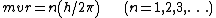The integer n is called the principal quantum number. The possible energies of the nonradiating states of the atom are given by Eq. (2).
(2)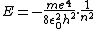Here 0 is the permittivity of free space, a constant included in order to give the correct units to the statement of Coulomb's law in SI units.

The same equation for the hydrogen atom's energy levels, except for some small but significant corrections, is obtained from the solution of the Schrödinger equation, as modified by W. Pauli, for the hydrogen atom. See Quantum numbers

The frequencies of electromagnetic radiation or light emitted or absorbed in transitions are given by Eq. (3),

(3)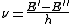where E and E are the energies of the initial and final states of the atom. Spectroscopists usually express their measurements in wavelength λ or in wave number &sgr; in order to obtain numbers of a convenient size. The wave number of a transition is shown in Eq. (4). (4)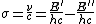If T = E(hc), then Eq. (5) results. Here T is called the spectral term. (5)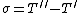The allowed terms for hydrogen, from Eq. (2), are given by Eq. (6).

(6)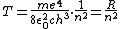The quantity R is the important Rydberg constant. Its value, which has been measured to a remarkable and rapidly improving accuracy, is related to the values of other well-known atomic constants, as in Eq. (6). See Rydberg constant

The effect of finite nuclear mass must be considered, since the nucleus does not actually remain at rest at the center of the atom. Instead, the electron and nucleus revolve about their common center of mass. This effect can be accurately accounted for and requires a small change in the value of the effective mass m in Eq. (6).

In addition to the circular orbits already described, elliptical ones are also consistent with the requirement that the angular momentum be quantized. A. Sommerfeld showed that for each value of n there is a family of n permitted elliptical orbits, all having the same major axis but with different eccentricities. Illustration a shows, for example, the Bohr-Sommerfeld orbits for n = 3. The orbits are labeled s, p, and d, indicating values of the azimuthal quantum number l = 0, 1, and 2. This number determines the shape of the orbit, since the ratio of the major to the minor axis is found to be n(l = 1). To a first approximation, the energies of all orbits of the same n are equal. In the case of the highly eccentric orbits, however, there is a slight lowering of the energy due to precession of the orbit (illus. b). According to Einstein's theory of relativity, the mass increases somewhat in the inner part of the orbit, because of greater velocity. The velocity increase is greater as the eccentricity is greater, so the orbits of higher eccentricity have their energies lowered more. The quantity l is called the orbital angular momentum quantum number or the azimuthal quantum number. See Relativity

#### Multielectron atoms

In attempting to extend Bohr's model to atoms with more than one electron, it is logical to compare the experimentally observed terms of the alkali atoms, which contain only a single electron outside closed shells, with those of hydrogen. A definite similarity is found but with the striking difference that all terms with l > 0 are double. This fact was interpreted by S. A. Goudsmit and G. E. Uhlenbeck as due to the presence of an additional angular momentum of (h2&pgr;) attributed to the electron spinning about its axis. The spin quantum number of the electron is s = .

The relativistic quantum mechanics developed by P. A. M. Dirac provided the theoretical basis for this experimental observation. See Electron spin

Implicit in much of the following discussion is W. Pauli's exclusion principle, first enunciated in 1925, which when applied to atoms may be stated as follows: no more than one electron in a multielectron atom can possess precisely the same quantum numbers. In an independent, hydrogenic electron approximation to multielectron atoms, there are 2n2 possible independent choices of the principal (n), orbital (l), and magnetic (ml, ms) quantum numbers available for electrons belonging to a given n, and no more. Here ml and ms refer to the quantized projections of l and s along some chosen direction. The organization of atomic electrons into shells of increasing radius (the Bohr radius scales as n2) follows from this principle. See Exclusion principle

The energy of interaction of the electron's spin with its orbital angular momentum is known as spin-orbit coupling. A charge in motion through either “pure” electric or “pure” magnetic fields, that is, through fields perceived as “pure” in a static laboratory, actually experiences a combination of electric and magnetic fields, if viewed in the frame of reference of a moving observer with respect to whom the charge is momentarily at rest. For example, moving charges are well known to be deflected by magnetic fields. But in the rest frame of such a charge, there is no motion, and any acceleration of a charge must be due to the presence of a pure electric field from the point of view of an observer analyzing the motion in that reference frame. See Relativistic electrodynamics

A spinning electron can crudely be pictured as a spinning ball of charge, imitating a circulating electric current. This circulating current gives rise to a magnetic field distribution very similar to that of a small bar magnet, with north and south magnetic poles symmetrically distributed along the spin axis above and below the spin equator. This representative bar magnet can interact with external magnetic fields, one source of which is the magnetic field experienced by an electron in its rest frame, owing to its orbital motion through the electric field established by the central nucleus of an atom. In multielectron atoms, there can be additional, though generally weaker, interactions arising from the magnetic interactions of each electron with its neighbors, as all are moving with respect to each other and all have spin. The strength of the bar magnet equivalent to each electron spin, and its direction in space are characterized by a quantity called the magnetic moment, which also is quantized essentially because the spin itself is quantized. Studies of the effect of an external magnetic field on the states of atoms show that the magnetic moment associated with the electron spin is equal in magnitude to a unit called the Bohr magneton.

The energy of the interaction between the electron's magnetic moment and the magnetic field generated by its orbital motion is usually a small correction to the spectral term, and depends on the angle between the magnetic moment and the magnetic field or, equivalently, between the spin angular momentum vector and the orbital angular momentum vector (a vector perpendicular to the orbital plane whose magnitude is the size of the orbital angular momentum). Since quantum theory requires that the quantum number j of the electron's total angular momentum shall take values differing by integers, while l is always an integer, there are only two possible orientations for s relative to l: s must be either parallel or antiparallel to l.

For the case of a single electron outside the nucleus, the Dirac theory gives Eq. (7)

(7)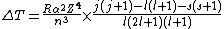for the spin-orbit correction to the spectral terms. Here α = e2/(2ε0hc) ≅ 1/137 is called the fine structure constant.

In atoms having more than one electron, this fine structure becomes what is called the multiplet structure. The doublets in the alkali spectra, for example, are due to spin-orbit coupling; Eq. (7), with suitable modifications, can still be applied.

When more than one electron is present in the atom, there are various ways in which the spins and orbital angular momenta can interact. Each spin may couple to its own orbit, as in the one-electron case; other possibilities are orbit-other orbit, spin-spin, and so on. The most common interaction in the light atoms, called LS coupling or Russell-Saunders coupling, is described schematically in Eq. (8).

(8)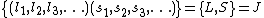This notation indicates that the l are coupled strongly together to form a resultant L, representing the total orbital angular momentum. The si are coupled strongly together to form a resultant S, the total spin angular momentum. The weakest coupling is that between L and S to form J, the total angular momentum of the electron system of the atom in this state.

Coupling of the LS type is generally applicable to the low-energy states of the lighter atoms. The next commonest type is called jj coupling, represented in Eq. (9).

(9)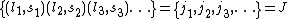Each electron has its spin coupled to its own orbital angular momentum to form a ji for that electron. The various ji are then more weakly coupled together to give J. This type of coupling is seldom strictly observed. In the heavier atoms it is common to find a condition intermediate between LS and jj coupling; then either the LS or jj notation may be used to describe the levels, because the number of levels for a given electron configuration is independent of the coupling scheme.

#### Nuclear magnetism and hyperfine structure

Most atomic nuclei also possess spin, but rotate about 2000 times slower than electrons because their mass is on the order of 2000 or more times greater than that of electrons. Because of this, very weak nuclear magnetic fields, analogous to the electronic ones that produce fine structure in spectral lines, further split atomic energy levels. Consequently, spectral lines arising from them are split according to the relative orientations, and hence energies of interaction, of the nuclear magnetic moments with the electronic ones. The resulting pattern of energy levels and corresponding spectral-line components is referred to as hyperfine structure. See Nuclear moments

Nuclear properties also affect atomic spectra through the isotope shift. This is the result of the difference in nuclear masses of two isotopes, which results in a slight change in the Rydberg constant. There is also sometimes a distortion of the nucleus, which can be detected by ultrahigh precision spectroscopy. See Molecular beams, Particle trap

In most cases, a common problem called Doppler broadening of the spectral lines arises, which can cause overlapping of spectral lines and make analysis difficult. The broadening arises from motion of the emitted atom with respect to a spectrometer. Several ingenious ways of isolating only those atoms nearly at rest with respect to spectrometric apparatus have been devised. The most powerful employ lasers and either involve saturation spectroscopy, utilizing a saturating beam and probe beam from the same tunable laser, or use two laser photons which jointly drive a single atomic transition and are generated in lasers so arranged that the first-order Doppler shifts of the photons cancel each other. See Doppler effect

It would be misleading to think that the most probable fate of excited atomic electrons consists of transitions to lower orbits, accompanied by photon emission. In fact, for at least the first third of the periodic table, the preferred decay mode of most excited atomic systems in most states of excitation and ionization is the electron emission process first observed by P. Auger in 1925 and named after him. For example, a singly charged neon ion lacking a 1s electron is more than 50 times as likely to decay by electron emission as by photon emission. In the process, an outer atomic electron descends to fill an inner vacancy, while another is ejected from the atom to conserve both total energy and momentum in the atom. The ejection usually arises because of the interelectron Coulomb repulsion. See Auger effect

#### Cooling and stopping atoms and ions

Despite impressive progress in reducing Doppler shifts and Doppler spreads, these quantities remain factors that limit the highest obtainable spectroscopic resolutions. The 1980s and 1990s saw extremely rapid development of techniques for trapping neutral atoms and singly charged ions in a confined region of space, and then cooling them to much lower temperatures by the application of laser-light cooling techniques. Photons carry not only energy but also momentum; hence they can exert pressure on neutral atoms as well as charged ions. See Laser cooling

Schemes have been developed to exploit these light forces to confine neutral atoms in the absence of material walls, whereas various types of so-called bottle configurations of electromagnetic fields developed earlier remain the technique of choice for similarly confining ions. Various ingenious methods have been invented to slow down and even nearly stop neutral atoms and singly charged ions, whose energy levels (unlike those of most more highly charged ions) are accessible to tunable dye lasers. These methods often utilize the velocity-dependent light pressure from laser photons of nearly the same frequency as, but slightly less energetic than, the energy separation of two atomic energy levels to induce a transition between these levels.

The magnetooptic trap combines optical forces provided by laser light with a weak magnetic field whose size goes through zero at the geometrical center of the trap and increases with distance from this center. The net result is a restoring force which confines sufficiently laser-cooled atoms near the center. Ingenious improvements have allowed cooling of ions to temperatures as low as 180 × 10-9 K.

For more highly ionized ions, annular storage rings are used in which radial confinement of fast ion beams (with speeds of approximately 10% or more of the speed of light) is provided by magnetic focusing. Two cooling schemes are known to work on stored beams of charged particles, the so-called stochastic cooling method and the electron cooling method. In the former, deviations from mean stored particle energies are electronically detected, and electronic “kicks” that have been adjusted in time and direction are delivered to the stored particles to compensate these deviations. In electron cooling, which proves to be more effective for stored heavy ions of high charge, electron beams prepared with a narrow velocity distribution are merged with the stored ion beams. When the average speeds of the electrons and the ions are matched, the Coulomb interaction between the relatively cold (low-velocity-spread) electrons and the highly charged ions efficiently transfers energy from the warmer ions, thereby reducing the temperature of the stored ions.

Site: Follow: Share:
Open / Close